# HashMap集合中的put方法，看完这一篇就够了Java技术迷 |152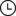2021-12-17 23:32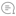0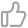00`作者 | 汪伟俊出品 | 公众号：Java技术迷（JavaFans1024）`

# HashMap底层数据结构

1.线性探测法2.伪随机数法3.链地址法

``static class Node<K,V> implements Map.Entry<K,V> {``    final int hash;``    final K key;``    V value;``    Node<K,V> next;``    Node(int hash, K key, V value, Node<K,V> next) {``        this.hash = hash;``        this.key = key;``        this.value = value;``        this.next = next;``    }``    public final K getKey()        { return key; }``    public final V getValue()      { return value; }``    public final String toString() { return key + "=" + value; }``    public final int hashCode() {``        return Objects.hashCode(key) ^ Objects.hashCode(value);``    }``    public final V setValue(V newValue) {``        V oldValue = value;``        value = newValue;``        return oldValue;``    }``    public final boolean equals(Object o) {``        if (o == this)``            return true;``        if (o instanceof Map.Entry) {``            Map.Entry<?,?> e = (Map.Entry<?,?>)o;``            if (Objects.equals(key, e.getKey()) &&``                Objects.equals(value, e.getValue()))``                return true;``        }``        return false;``    }``}``

``transient Node<K,V>[] table;``

# put方法的执行流程

``public static void main(String[] args) {``    Map<String, String> map = new HashMap<>();``    map.put("name", "zs");``    map.put("age", "20");``    map.put("name", "lisi");``    map.forEach((k, v) -> {``        System.out.println(k + "--" + v);``    });``}``

``public HashMap() {``    this.loadFactor = DEFAULT_LOAD_FACTOR; // all other fields defaulted``}``

``public V put(K key, V value) {``    return putVal(hash(key), key, value, false, true);``}``

put方法又调用了putVal方法，并传入了key的hash，key，value等等参数，所以先来计算key的hash：

``static final int hash(Object key) {``    int h;``    return (key == null) ? 0 : (h = key.hashCode()) ^ (h >>> 16);``}``

``final V putVal(int hash, K key, V value, boolean onlyIfAbsent,``               boolean evict) {``    Node<K,V>[] tab; Node<K,V> p; int n, i;``    if ((tab = table) == null || (n = tab.length) == 0)``        n = (tab = resize()).length;``    if ((p = tab[i = (n - 1) & hash]) == null)``        tab[i] = newNode(hash, key, value, null);``    else {``        Node<K,V> e; K k;``        if (p.hash == hash &&``            ((k = p.key) == key || (key != null && key.equals(k))))``            e = p;``        else if (p instanceof TreeNode)``            e = ((TreeNode<K,V>)p).putTreeVal(this, tab, hash, key, value);``        else {``            for (int binCount = 0; ; ++binCount) {``                if ((e = p.next) == null) {``                    p.next = newNode(hash, key, value, null);``                    if (binCount >= TREEIFY_THRESHOLD - 1) // -1 for 1st``                        treeifyBin(tab, hash);``                    break;``                }``                if (e.hash == hash &&``                    ((k = e.key) == key || (key != null && key.equals(k))))``                    break;``                p = e;``            }``        }``        if (e != null) { // existing mapping for key``            V oldValue = e.value;``            if (!onlyIfAbsent || oldValue == null)``                e.value = value;``            afterNodeAccess(e);``            return oldValue;``        }``    }``    ++modCount;``    if (++size > threshold)``        resize();``    afterNodeInsertion(evict);``    return null;``}``

``final Node<K,V>[] resize() {``    Node<K,V>[] oldTab = table;``    int oldCap = (oldTab == null) ? 0 : oldTab.length;``    int oldThr = threshold;``    int newCap, newThr = 0;``    if (oldCap > 0) {``        if (oldCap >= MAXIMUM_CAPACITY) {``            threshold = Integer.MAX_VALUE;``            return oldTab;``        }``        else if ((newCap = oldCap << 1) < MAXIMUM_CAPACITY &&``                 oldCap >= DEFAULT_INITIAL_CAPACITY)``            newThr = oldThr << 1; // double threshold``    }``    else if (oldThr > 0) // initial capacity was placed in threshold``        newCap = oldThr;``    else {               // zero initial threshold signifies using defaults``        newCap = DEFAULT_INITIAL_CAPACITY;``        newThr = (int)(DEFAULT_LOAD_FACTOR * DEFAULT_INITIAL_CAPACITY);``    }``    if (newThr == 0) {``        float ft = (float)newCap * loadFactor;``        newThr = (newCap < MAXIMUM_CAPACITY && ft < (float)MAXIMUM_CAPACITY ?``                  (int)ft : Integer.MAX_VALUE);``    }``    threshold = newThr;``    @SuppressWarnings({"rawtypes","unchecked"})``        Node<K,V>[] newTab = (Node<K,V>[])new Node[newCap];``    table = newTab;``    if (oldTab != null) {``        for (int j = 0; j < oldCap; ++j) {``            Node<K,V> e;``            if ((e = oldTab[j]) != null) {``                oldTab[j] = null;``                if (e.next == null)``                    newTab[e.hash & (newCap - 1)] = e;``                else if (e instanceof TreeNode)``                    ((TreeNode<K,V>)e).split(this, newTab, j, oldCap);``                else { // preserve order``                    Node<K,V> loHead = null, loTail = null;``                    Node<K,V> hiHead = null, hiTail = null;``                    Node<K,V> next;``                    do {``                        next = e.next;``                        if ((e.hash & oldCap) == 0) {``                            if (loTail == null)``                                loHead = e;``                            else``                                loTail.next = e;``                            loTail = e;``                        }``                        else {``                            if (hiTail == null)``                                hiHead = e;``                            else``                                hiTail.next = e;``                            hiTail = e;``                        }``                    } while ((e = next) != null);``                    if (loTail != null) {``                        loTail.next = null;``                        newTab[j] = loHead;``                    }``                    if (hiTail != null) {``                        hiTail.next = null;``                        newTab[j + oldCap] = hiHead;``                    }``                }``            }``        }``    }``    return newTab;``}``

``else {               // zero initial threshold signifies using defaults``    newCap = DEFAULT_INITIAL_CAPACITY;``    newThr = (int)(DEFAULT_LOAD_FACTOR * DEFAULT_INITIAL_CAPACITY);``}``

``threshold = newThr;``@SuppressWarnings({"rawtypes","unchecked"})``Node<K,V>[] newTab = (Node<K,V>[])new Node[newCap];``table = newTab;``

``if ((p = tab[i = (n - 1) & hash]) == null)``    tab[i] = newNode(hash, key, value, null);``

``Node<K,V> newNode(int hash, K key, V value, Node<K,V> next) {``    return new Node<>(hash, key, value, next);``}``

``++modCount;``if (++size > threshold)``    resize();``afterNodeInsertion(evict);``

``if ((p = tab[i = (n - 1) & hash]) == null)``    tab[i] = newNode(hash, key, value, null);``

``Node<K,V> e; K k;``if (p.hash == hash &&``    ((k = p.key) == key || (key != null && key.equals(k))))``    e = p;``

``if (e != null) { // existing mapping for key``    V oldValue = e.value;``    if (!onlyIfAbsent || oldValue == null)``        e.value = value;``    afterNodeAccess(e);``    return oldValue;``}``

# 总结

HashMap的总容量一定是2的幂次方，即使通过构造函数传入一个不是2的幂次方的容量，HashMap也会将其扩充至与其最接近的2的幂次方的值；比如传入总容量为10，则HashMap会自动将容量扩充至16若是调用HashMap的无参构造方法，则将在第一次执行put方法时初始化一个总容量为16，实际可用容量为12的Node数组当实际容量超过阈值时，HashMap会进行扩容，扩容至原容量的2倍HashMap的put方法执行流程：首先判断当前table是否为空，若为空，则初始化，若不为空，则根据key的hash计算得到插入位置，再判断该位置是否有元素，若无元素，则直接插入，若有元素，则判断原位置数据的hash值与待插入数据的hash值是否相同，若相同，则继续比较值，若值不同，则创建一个新的Node节点，并使用尾插法将其插入到原数据的节点后面形成链表，若值相同，则采用待插入数据的值覆盖原数据的值，并返回原数据的值HashMap采用链地址法解决hash冲突，所以当某个链表的长度大于8，并且table数组的长度大于64，则当前链表会被转换为红黑树，若table数组的长度尚未达到64，则进行扩容；当链表长度小于6，则会将红黑树转回链表因为HashMap会根据key的hash值计算插入位置，所以key的数据类型一定要重写hashCode方法，否则会出现两个相同的key结果hash值不相同的情况，也需要重写equals方法，否则equals方法将比较的是地址值

`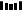往期推荐1、致歉！抖音Semi Design承认参考阿里Ant Design2、对比7种分布式事务方案，还是偏爱阿里开源的Seata，真香！3、Redis存储结构体信息，选hash还是string？4、扫盲 docker 常用命令5、最全分布式Session解决方案6、21 款 yyds 的 IDEA插件7、真香！用 IDEA 神器看源码，效率真高！点分享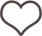点收藏点点赞点在看`00

暂无评论~~
Ctrl+Enter